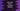# Constants defined in dart-math library### Constants defined in dart:math library:

dart:math library provides a couple of mathematical constants, functions and a random number generator. In this post, we will check the constants of dart:math with example.

### Constants in dart:math :

Following are the constants defined in dart:math :

#### e → const double:

This is the base of the natural algorithm.

#### ln2 → const double:

This is the natural logarithm of 2.

#### ln10 → const double:

Natural logarithm of 10.

#### log2e → const double:

Base 2 logarithm of e.

#### log10e → const double:

Base 10 logarithm of e.

Value of PI.

#### sqrt1_2 → const double:

Square root of 1/2

#### sqrt2 → const double:

Square root of 2.

Let’s take a look at the example below:

``````import 'dart:math';

void main() {
print("e : \${e}");
print("ln2 : \${ln2}");
print("ln10 : \${ln10}");
print("log2e : \${log2e}");
print("log10e : \${log10e}");
print("pi : \${pi}");
print("sqrt1_2 : \${sqrt1_2}");
print("sqrt2 : \${sqrt2}");
}``````

This program is printing the values of all constants of dart:math.

``````e : 2.718281828459045
ln2 : 0.6931471805599453
ln10 : 2.302585092994046
log2e : 1.4426950408889634
log10e : 0.4342944819032518
pi : 3.141592653589793
sqrt1_2 : 0.7071067811865476
sqrt2 : 1.4142135623730951``````